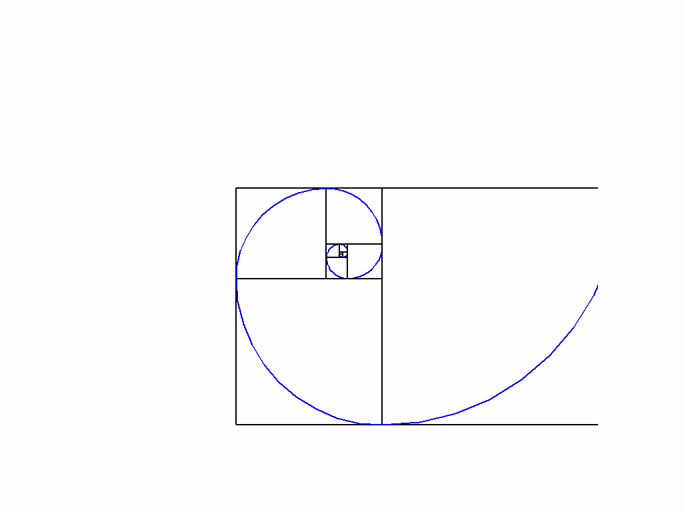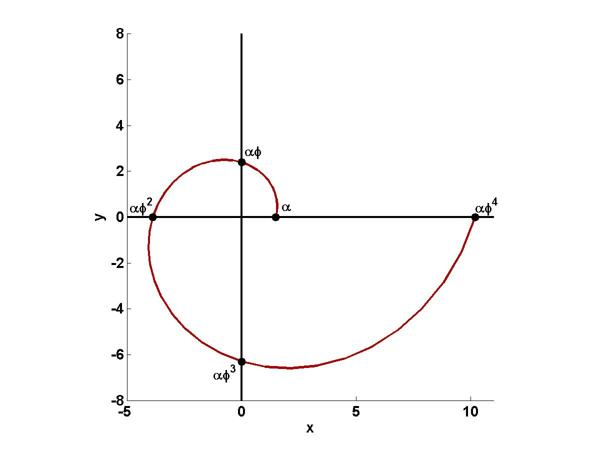# Golden Spiral

A Golden Spiral is simulated by a continuously expanding sequence of golden rectangles and inscribed quarter circles.### Contents

#### Golden Rectangles

We begin with an animated .gif of an imitation Golden Spiral. You can see that removing a square from a golden rectangle leaves a smaller rectangle with the same shape. Connecting inscribed quarter circles produces a continuously expanding spiral. The aspect ratio of the rectangles is the golden ratio.

$$\phi = \frac{1 + \sqrt{5}}{2}$$

A MATLAB function for generating these expanding golden rectangles and quarter circles is given at the end of this blog post, but this is not a true Golden Spiral.

#### True Golden Spiral

A logarithmic spiral is a curve given in polar coordinates by

$$r = \alpha e^{\lambda \theta}$$

The angular coordinate $\theta$ must be multi-valued as the point circles around the origin multiple times. We get the particular logarithmic spiral known as the Golden Spiral by involving the golden ratio and setting

$$\lambda = \frac{2}{\pi}{\ln{\phi}}$$

Then the radius is scaled powers of $\phi$, with integer powers as $\theta$ crosses the cartesian axes.

$$r = \alpha \phi^{\frac{2}{\pi} \theta}$$

Here is the plot for $0 \le \theta \le 2 \pi$.I do not see any obvious analytic way to specify the scale factor $\alpha$. By taking $\alpha$ = 1.48699214 the numeric values generated by the logarithmic Golden Spiral approach those generated by golden rectangles and inscribed quarter circles as the angle $\theta$ gets large.

#### golden_spiral.m

function golden_spiral
% GOLDEN_SPIRAL  Explosion of golden rectangles.
%    GOLDEN_SPIRAL  Constructs a continuously expanding sequence
%    of golden rectangles and inscribed quarter circles.
%   Copyright 2013 Cleve Moler
%   Copyright 2013 The MathWorks, Inc.
   % Initialize_variables
   % Golden ratio
phi = (1+sqrt(5))/2;
   % Control speed of zoom
n = 256;
f = phi^(1/n);
   % Scaling
a = 1;
s = phi;
t = 1/(phi+1);
   % Centers
x = 0;
y = 0;
   % A square
us = [-1 1 1 -1 -1];
vs = [-1 -1 1 1 -1];
   % Four quarter circles
theta = 0:pi/20:pi/2;
u1 = 2*cos(theta) - 1;
v1 = 2*sin(theta) - 1;
u2 = 2*cos(theta+pi/2) + 1;
v2 = 2*sin(theta+pi/2) - 1;
u3 = 2*cos(theta+pi) + 1;
v3 = 2*sin(theta+pi) + 1;
u4 = 2*cos(theta-pi/2) - 1;
v4 = 2*sin(theta-pi/2) + 1;
   initialize_graphics
   % Loop
   k = 0;
while get(klose,'value') == 0
if mod(k,n) == 0
power
switch mod(k/n,4)
case 0, right
case 1, up
case 2, left
case 3, down
end
end
zoom
k = k+1;
end
pause(1)
close(gcf)
% ------------------------------------
   function power
a = s;
s = phi*s;
t = phi*t;
end % power
% ------------------------------------
   function zoom
axis(f*axis)
drawnow
end % zoom
% ------------------------------------
   function right
x = x + s;
y = y + t;
line(x+a*us,y+a*vs,'color','black')
line(x+a*u4,y+a*v4)
end % right
% ------------------------------------
   function up
y = y + s;
x = x - t;
line(x+a*us,y+a*vs,'color','black')
line(x+a*u1,y+a*v1)
end % up
% ------------------------------------
   function left
x = x - s;
y = y - t;
line(x+a*us,y+a*vs,'color','black')
line(x+a*u2,y+a*v2)
end % left
% ------------------------------------
   function down
y = y - s;
x = x + t;
line(x+a*us,y+a*vs,'color','black')
line(x+a*u3,y+a*v3)
end % down
% ------------------------------------
   function initialize_graphics
clf reset
'name','Golden Spiral')
shg
axes('position',[0 0 1 1])
axis(3.5*[-1 1 -1 1])
axis square
axis off
line(us,vs,'color','black')
line(u3,v3)
klose = uicontrol('units','normal','position',[.04 .04 .12 .04], ...
'style','toggle','string','close','vis','on');
drawnow
end % initialize graphics
end % golden_spiral

Published with MATLAB® R2013b

|